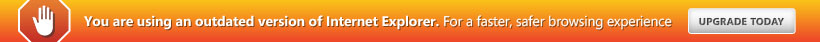PHS High School Curriculum

• Th12 10, 2013
• 66 views

To ensure all-round learning and development for each student, the high school study program at PHS is distributed by grade levels as follows:

PHÂN PHỐI CHƯƠNG TRÌNH – TRUNG HỌC PHS

No. Subjects / Grades 6 7 8 9 10 11 12
1 Văn x x x x x x x
2 Toán x x x x x x x
3 Vật lý x x x x x x x
4 Hóa học     x x x x x
5 Sinh học x x x x x x x
6 CNTT x x x x x x x
7 Nghề phổ thông           x
8 Công nghệ x x x x x x x
9 Lịch sử x x x x x x x
10 Địa lý x x x x x x x
11 Giáo dục công dân x x x x x x x
12 Giáo dục quốc phòng         x x x
13 Mỹ thuật x x x x
14 Thể dục x x x x x x x
15 Thể thao x x x x x x
16 Nhạc x x x
17 Tiếng Anh x x x x x x x
18 Advanced English x x x x x x x
19 Luyện thi IELTS         x x x
20 Maths x x x x x x
21 Sciences x x x x x x
22 Public speaking x x x x
23 World Culture x x x
24 Tourism       x x